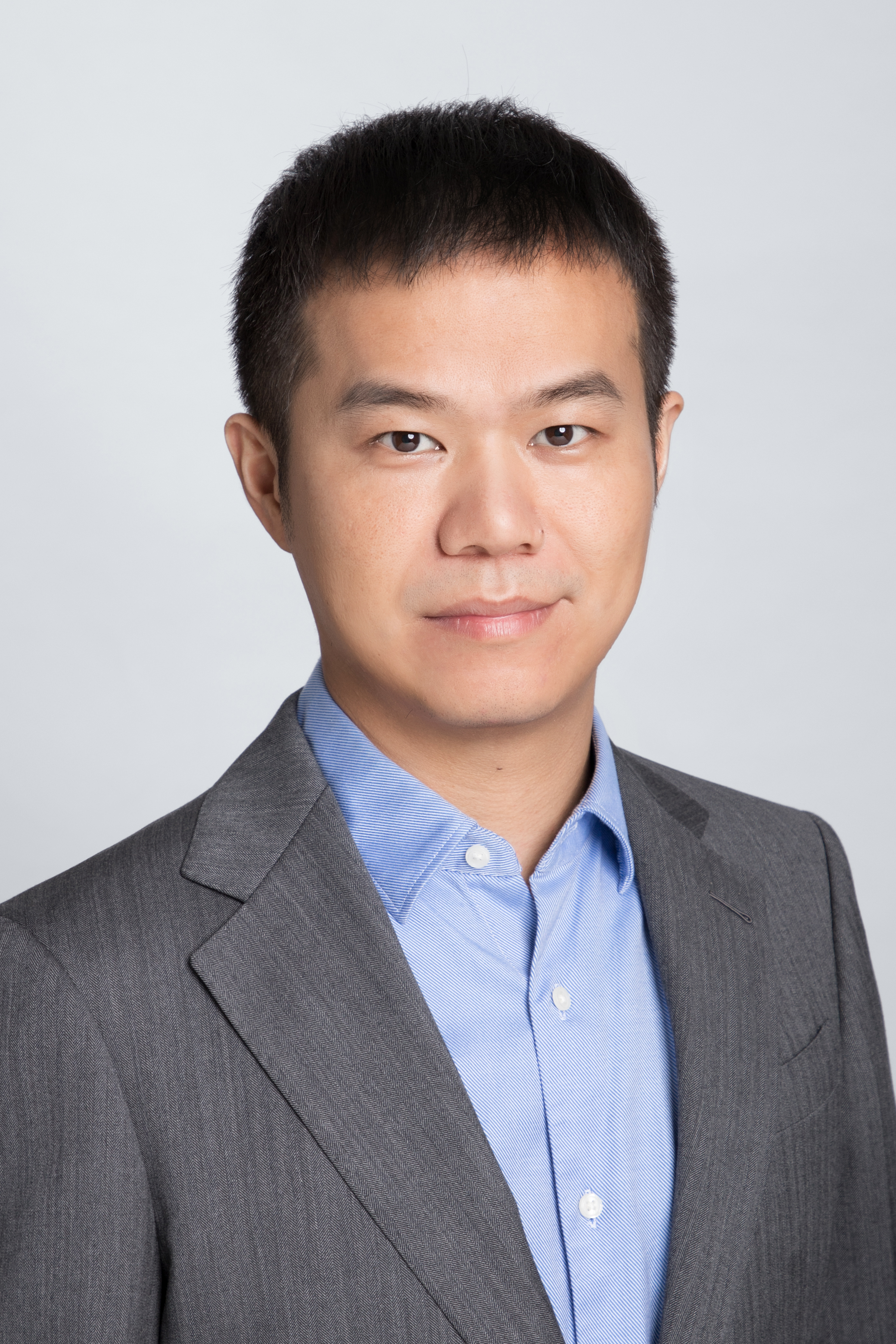## Jiang YANG

Associate Professor Department of Mathematics

Education：

Ph.D., Hong Kong Baptist University, Mathematics, 2011.6-2014.7, Advisor: Prof. Tao TANG.

B.S., Zhejiang University, Mathematics, 2006.9-2010-7

Professional Experience:

Associate Professor, Southern University of Science and Technology, Department of Mathematics, 2018.2 - present

Assistant Professor, Southern University of Science and Technology, Department of Mathematics, 2017.7 - 2018.1

Postdoctoral Research Scientist, Columbia University, Applied Physics and Applied Mathematics, 2015.8 - 2017.6, Mentor: Prof. Qiang Du.

Research Associate, Pennsylvania State University, Department of Mathematics, 2014.8 - 2015.7, Mentor: Prof. Qiang Du.

## Research

◆ Numerical Partial Differential Equations

◆ Numerical solutions of phase-field models and their applications

◆ Numerical solutions of nonlocal models and their applications

## Teaching

Fall 2019 MA305: Numerical Analysis, SUSTech, China

Spring 2019 MA325: Numerical PDEs, SUSTech, China

Fall 2018 MA305: Numerical Analysis, SUSTech, China

Fall 2018 MA130A: Linear algebra I A, SUSTech, China

Spring 2018 MA325: Numerical PDEs, SUSTech, China

Fall 2017 MA305: Numerical Analysis, SUSTech, China

Fall 2016 MA366: Ordinary Differential Equations , Purdue University, USA

J. Shen, J. Xu, and J. Yang, A new class of efficient and robust energy stable schemes for gradient flows, SIAM Rev., 61-3 (2019), pp. 474-506.

L.F. Li, S. S. Luo, X.C. Tai and J. Yang, A Variational Convex Hull Algorithm, Seventh International Conference on Scale Space and Variational Methods in Computer Vision, 2019, organized by Microsoft Corporation.

Tao Tang and Jiang Yang, Computing the Maximal Eigenpairs of Large Size Tridiagonal Matrices with O(1) Number of Iterations, Numer. Math. Theor. Meth. Appl., 11 (2018), pp. 877-894.

Q. Du, Y. Tao, X. Tian and J. Yang, Asymptotically compatible discretization of multidimensional nonlocal diffusion models and approximation of nonlocal Green's functions, IMA J. Numer. Anal., 39(2) (2019), 607-625.

J. Shen, J. Xu, and J. Yang, The scalar auxiliary variable (SAV) approach for gradient flows, J. Comput. Phys., 353 (2018), 407-416.

Q. Du, J. Yang, and W. Zhang, Numerical analysis on the uniform \$L^p\$-stability of Allen-Cahn equations, Int. J. Numer. Anal. Mod., 15(1-2) (2018), 213-227.

T. Hou, T. Tang and J. Yang, Numerical analysis of fully discretized Crank--Nicolson scheme for fractional-in-space Allen-Cahn equations, J. Sci. Comput., 72(3) (2017), 1214-1231.

Q. Du and J. Yang, Fast and Accurate Implementation of Fourier Spectral Approximations of Nonlocal Diffusion Operators and its Applications, J. Comput. Phys., 332 (2017), 118-134.

Q. Du, J. Yang and Zhi Zhou, Analysis of a nonlocal-in-time parabolic equations, Dis. Cont. Dyn. Sys. B, 22(2) (2017), 339-368.

W. Zhang, J. Yang, J. Zhang, and Q. Du, Artificial boundary conditions for nonlocal heat equations on unbounded domain, Comm. Comp. Phys., 21(1) (2017), 16-39.

J. Shen, T. Tang and J. Yang, On the maximum principle preserving schemes for the generalized Allen-Cahn equation, Comm. Math. Sci., 14(6) (2016), 1517-1534.

T. Tang and J. Yang, Implicit-explicit scheme for the Allen-Cahn equation preserves the maximum principle, J. Comput. Math., 34(5) (2016), 471-481.

Q. Du, Y. Tao, X. Tian and J. Yang, Robust a posteriori stress analysis for approximations of nonlocal models via nonlocal gradients, Comp. Meth. Appl. Mech. Eng. 310 (2016), 605-627.

Q. Du and J. Yang, Asymptotically compatible Fourier spectral approximations of nonlocal Allen-Cahn equations, SIAM J. Numer. Anal., 54(3) (2016), 1899-1919.

X. Feng, T. Tang and J. Yang, Long time numerical simulations for phase-field problems using p-adaptive spectral deferred correction methods, SIAM J. Sci. Comput. 37 (2015), A271-A294.

X. Feng, T. Tang and J. Yang, Stabilized Crank-Nicolson/Adams-Bashforth schemes for phase field models, East Asian Journal on Applied Mathematics, 3 (2013), pp. 59-80.

X. Feng, H. Song, T. Tang, and J. Yang, Nonlinear stability of the implicit-explicit methods for the Allen-Cahn equation, Inverse Problems and Imaging, Volume 7 (2013), pp. 679 - 695.

• 南科大研究生招生

• 博士后招聘